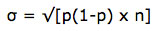SEARCH HOMEMath Central Quandaries & QueriesQuestion from silvestre, a student: A large company finds that approximately 17% of all purchases are returned for credit. If the company sells 100,000 different items this year, about how many items will be returned? Find the Standard Deviation.Let's look at a similar example: The number of times an employee was observed to be working during the day was 36 out of a total of 50 random observations. Find the standard deviation.

We can use the formulawhere σ is standard deviation, p is the proportion and n is the sample size.

In this case p=36/50=0.72 and n=50

σ = √(0.72)(1-0.72)(50) = √(0.72)(0.28)(50) = √10.08 = 3.2

I hope this helps,

JaniceMath Central is supported by the University of Regina and The Pacific Institute for the Mathematical Sciences.